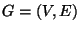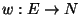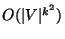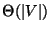Next: MINIMUM VERTEX K-CUT Up: Cuts and Connectivity Previous: MINIMUM NETWORK INHIBITION ON   Index

### MINIMUM K-CUT

• INSTANCE: Graph, a weight function, and an integer.

• SOLUTION: A partition of V into k disjoint sets.

• MEASURE: The sum of the weight of the edges between the disjoint sets, i.e.,• Good News: Approximable within.

• Comment: Solvable in polynomial timefor fixed k . If the sets in the partition are restricted to be of equal size, the problem is approximable within. If the sets in the partition are restricted to be of specified sizes and the weight function satisfies the triangle inequality, the problem is approximable within 3 for any fixed k . The unweighted problem admits a PTAS if every vertex has degree.

Viggo Kann
2000-03-20